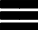Krivalar TutorialsKrivalar Tutorials

# Java if else in Programming

## if-else statement

if else statement is used to execute 2 different block of statements based on whether if condition returns true or false

## Example of if-else statement

``````public class IfElse {
public static void main(String a[]){
int i=3+3-6+8-9+4-6;
if(i>1){
System.out.println("Just do it");
}
else{
System.out.println("Just leave it");
}
}
}``````
Above code will print "Just do it" if the value of i is greater than 1. Otherwise, code prints "Just Leave it". In this case, value of i is -3 which is less than 1 and hence prints "Just Leave it"

## if-else-if statement

Example of if-else-if statement:
``````
public class IfElseIf {
public static void main(String a[]){
int marks=75;
if(marks>=90){
System.out.println("Excellent");
}
else if(marks>=80){
System.out.println("Good");
}
System.out.println("Nothing");
}
}``````
Above code will print "Excellent" if the value of marks is greater than 90. Otherwise, if marks is greater than 80, "Good" will be printed. If the other two conditions returns false, then it prints "Nothing". In this case, since marks=75,it prints "Nothing"